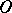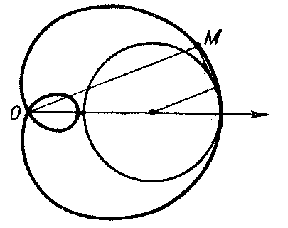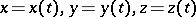# Pedal curve

of a curvewith respect to a pointThe set of bases to the perpendiculars dropped from the pointto the tangents to the curve. For example, the Pascal limaçon is the pedal of a circle with respect to the point(see Fig.). The pedal (curve) of a plane curverelative to the coordinate origin isFigure: p071950a

The equation for the pedal of a curvein space relative to the origin isThe antipedal of a curvewith respect to a pointis the name given to the curve with as pedal, with respect to the point, the curve.

The pedal of a surface with respect to a pointis the set of bases to the perpendiculars dropped from the pointto the tangent planes to the surface. The equation for the pedal of a surfacewith respect to the coordinate origin iswhere